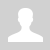Binary logic. Truth tables

11.3.3.3 build truth tables AND, OR, NOT, NAND, NOR, XOR

Binary logic. Truth tables

Binary logic

At the most elementary level, an elecrtonic device can only recognise the presence or absence of current or voltage. Either electricity is present or isn't. This is a switch - on or off, True or False, 1 or 0. With a computer's semiconductor, the voltage at the input and output terminals is measured and is either high or low; 1 or 0. Computers comprise billions of these switches and manipulating these sequences of Ons and Offs can change individual bits.

Electronic logic gates can take one or more inputs and produce a single output. This output can become the input to another gate and a complicated cascaded sequence of logic gates can be implemented to from a circuit in, for example, the CPU.

Logic gates and truth tables

There are a number of different logic gates that are each designed to perform a different operation in terms of output. These are: NOT, AND, OR, XOR, NAND and NOR gates.

source of gif: vivaxsolutions.com

What is a truth table?

A truth table is a table representing the output boolean values of a logical expression based on their entries. The table thus presents all the possible combinations of the input logical variables (generally 0 / FALSE and 1 / TRUE) and the result of the equation as output.

NOT gate

The NOT gate is represented by the symbol below and inverts the input. The small circle denotes an inverted input.

Using 1s and 0s as input to a gate, its operation can summarised in the form of a truth table.

NOR gate Truth tableQ = NOT A

Input A Output Q
1 0
0 1

AND gate

AND gate Truth table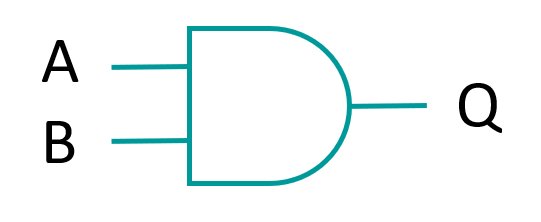Q = A AND B

Input A Input B Output Q
1 1 1
1 0 0
0 1 0
0 0 0

The boolean expression for AND is written: Q = A * B where * represents AND.

The truth table reflects the fundamental property of the AND gate: the output of A AND B is 1 only if input A and input B are both 1.

OR gate

OR gate Truth table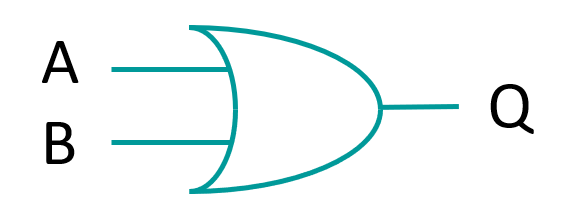Q = A OR B

Input A Input B Output Q
1 1 1
1 0 1
0 1 1
0 0 0

The boolean expression for OR is written: Q = A + B where + represents OR.

The truth table reflects the fundamental property of the OR gate: the output of A OR B is 1 if input A or input B is 1.

Creating logic gate circuits

Multiple logic gates can be connected to produce an output based on multiple inputs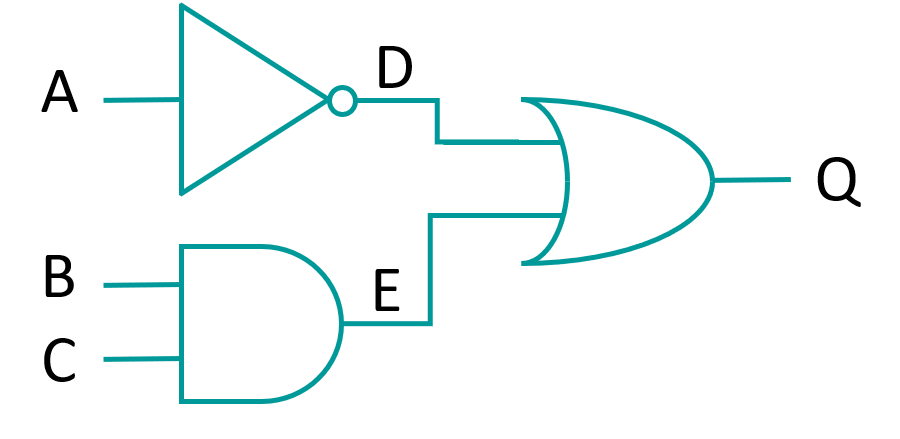This citcuit can be represented by Q = (NOT A)  OR (B AND C) or Q = -A + (B * C) and shown using the truth table below:

Input A Input B Input C D = NOT A E = B AND C Q = D OR E
1 1 1 0 1 1
1 1 0 0 0 0
1 0 1 0 0 0
1 0 0 0 0 0
0 1 1 1 1 1
0 1 0 1 0 1
0 0 1 1 0 1
0 0 0 1 0 1

XOR gate

The XOR (ex-or) gate stands for exclusive OR, meaning that the output will be true if one or other input is true, but not both. Compare this to the OR gate? Which outputs true if either or both inputs are true.

XOR gate Truth table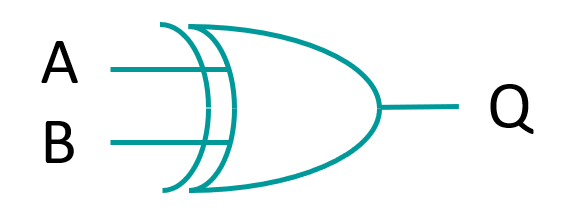Q = A XOR B

Input A Input B Output Q
1 1 0
1 0 1
0 1 1
0 0 0

The Boolean algebraic expression is written: Q = A ⊕ B where ⊕ the represents XOR, and is the equivalent of Q = (A * (-B)) + ((-A) * B). This gate similar to the OR gate but excludes the condition where A and B are both true.

NAND gate

The NAND gate is an combination of the AND and NOT gates, which inverts the output of the AND gate. Having a single type of NAND gate that can that can perform two separate functions can help to reduce development costs if a NAND gate is cheaper than separate AND and NOT gates.

NAND gate Truth table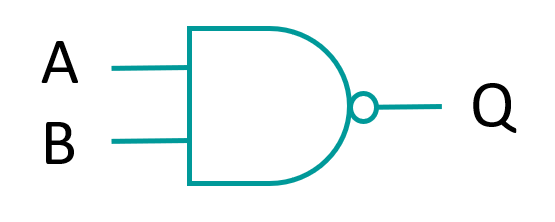Q = NOT (A AND B)

Input A Input B Output Q
1 1 0
1 0 1
0 1 1
0 0 1

The Boolean algebraic expression id written:.

NOR gate

NOR gate Truth table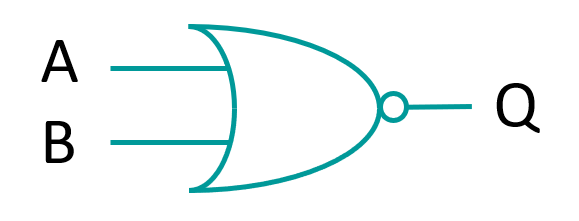Q = NOT (A OR B)

Input A Input B Output Q
1 1 0
1 0 0
0 1 0
0 0 1

The Boolean algebraic expression is written:. This gate only produces an output of true when both inputs are false.

Questions:

1) Explain what is boolean data type.

2) Build from memory the truth tables AND, OR, NOT, NAND, NOR, XOR.

3) What difference between AND and OR?

4) What difference between AND and NAND?

5) What difference between OR and XOR?

Exercises:

Ex. 1

Ex. 2

Test "Boolean logic"

Ex. 3

Ex. 4

Ex. 5

Ex. 6

Logic gates - Inteactive QUIZ

Exam questions:

Draw a truth table for the following circuit (Marks: ):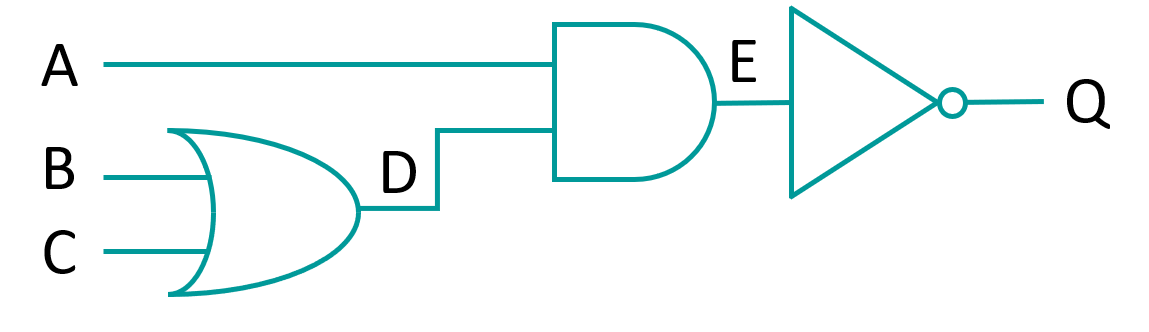• Input A Input B Input C D = B OR C E = A AND D Q = NOT E
1 1 1 1 1 0
1 1 0 1 1 0
1 0 1 1 1 0
1 0 0 0 0 1
0 1 1 1 0 1
0 1 0 1 0 1
0 0 1 1 0 1
0 0 0 0 0 1
Категория: Boolean logic | Добавил: bzfar77 (11.09.2020)
Просмотров: 727 | Теги: and, Or, Nor, Logic, logic gate, nand, XOR, not, Boolean, truth tables | Рейтинг: 5.0/1
 Всего комментариев: 0